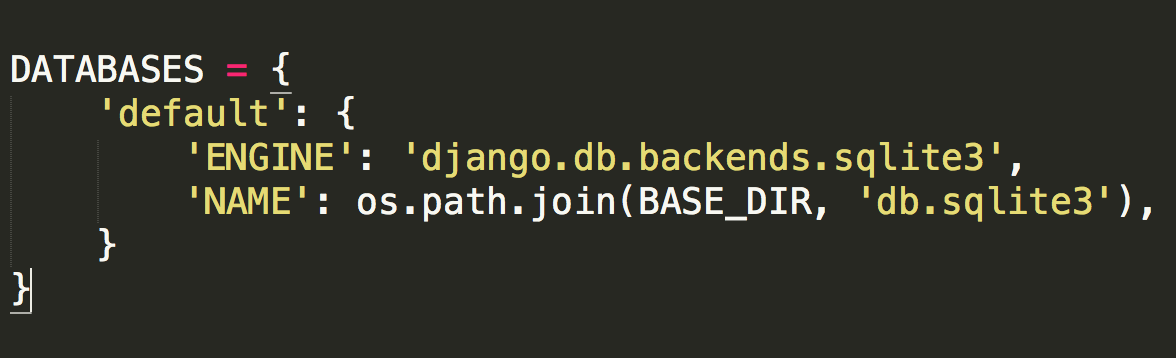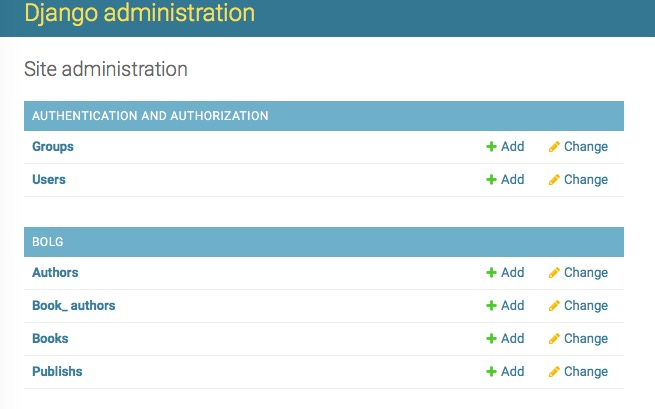# 一、Models

## 1、数据库的配置

1.1 django默认支持sqlite，mysql, oracle,postgresql数据库。

<1> sqlite

django默认使用sqlite的数据库，默认自带sqlite的数据库驱动 , 引擎名称：django.db.backends.sqlite3

<2> mysql

引擎名称：django.db.backends.mysql

#### 1.2 mysql驱动程序

•    MySQLdb(mysql python)
•    mysqlclient
•    MySQL
•    PyMySQL(纯python的mysql驱动程序)

#### 1.3 在django的项目中会默认使用sqlite数据库，在settings里有如下设置：DATABASES = {
# 'default': {
#     'ENGINE': 'django.db.backends.sqlite3',
#     'NAME': os.path.join(BASE_DIR, 'db.sqlite3'),
# }
'default': {
'ENGINE': 'django.db.backends.mysql',
'NAME':'django',  #库名
'USER': 'django',  #用户名
'HOST': '192.168.10.146', #ip
'PORT': '3306',  #端口
}
}NAME即数据库的名字，在mysql连接前该数据库必须已经创建，而上面的sqlite数据库下的db.sqlite3则是项目自动创建

import pymysql
pymysql.install_as_MySQLdb()

## 2、ORM(对象关系映射)

django为使用一种新的方式，即：关系对象映射（Object Relational Mapping，简称ORM）。

django中遵循 Code Frist 的原则，即：根据代码中定义的类来自动生成数据库表。

### 2.1、表(模型)的创建：from django.db import models<br>
class Publisher(models.Model):
name = models.CharField(max_length=30, verbose_name="名称")
city = models.CharField('城市',max_length=60)
state_province = models.CharField(max_length=30)
country = models.CharField(max_length=50)
website = models.URLField()

class Meta:
verbose_name = '出版商'
verbose_name_plural = verbose_name

def __str__(self):
return self.name

class Author(models.Model):
name = models.CharField(max_length=30)
def __str__(self):
return self.name

class AuthorDetail(models.Model):
sex = models.BooleanField(max_length=1, choices=((0, '男'),(1, '女'),))
email = models.EmailField()
birthday = models.DateField()
author = models.OneToOneField(Author)

class Book(models.Model):
title = models.CharField(max_length=100)
authors = models.ManyToManyField(Author)
publisher = models.ForeignKey(Publisher)
publication_date = models.DateField()
price=models.DecimalField(max_digits=5,decimal_places=2,default=10)
def __str__(self):
return self.title

from django.db import models

class Book(models.Model):

name=models.CharField(max_length=32)
price=models.IntegerField()
post_data=models.DateField()class publish(models.Model):
    name=models.CharField(max_length=32)    city=models.CharField(max_length=16)
class Author(models.Model):     name = models.CharField(max_length=32)

<1>  每个数据模型都是django.db.models.Model的子类，它的父类Model包含了所有必要的和数据库交互的方法。并提供了一个简介漂亮的定义数据库字段的语法。

<2>  每个模型相当于单个数据库表（多对多关系例外，会多生成一张关系表），每个属性也是这个表中的字段。属性名就是字段名，它的类型（例如CharField）相当于数据库的字段类型

（例如varchar）。大家可以留意下其它的类型都和数据库里的什么字段对应。

<3>  模型之间的三种关系：一对一，一对多，多对多。

一对一：实质就是在主外键（author_id就是foreign key）的关系基础上，给外键加了一个UNIQUE＝True的属性；

一对多：就是主外键关系；（foreign key）

多对多：(ManyToManyField) 自动创建第三张表(当然我们也可以自己创建第三张表：两个foreign key)<1> CharField
#字符串字段, 用于较短的字符串.
#CharField 要求必须有一个参数 maxlength, 用于从数据库层和Django校验层限制该字段所允许的最大字符数.

<2> IntegerField
#用于保存一个整数.

<3> FloatField
# 一个浮点数. 必须 提供两个参数:
#
# 参数    描述
# max_digits    总位数(不包括小数点和符号)
# decimal_places    小数位数
# 举例来说, 要保存最大值为 999 (小数点后保存2位),你要这样定义字段:
#
# models.FloatField(..., max_digits=5, decimal_places=2)
# 要保存最大值一百万(小数点后保存10位)的话,你要这样定义:
#
# models.FloatField(..., max_digits=19, decimal_places=10)

<4> AutoField
# 一个 IntegerField, 添加记录时它会自动增长. 你通常不需要直接使用这个字段;
# 自定义一个主键：my_id=models.AutoField(primary_key=True)
# 如果你不指定主键的话,系统会自动添加一个主键字段到你的 model.

<5> BooleanField
# A true/false field. admin 用 checkbox 来表示此类字段.

<6> TextField
# 一个容量很大的文本字段.

<7> EmailField
# 一个带有检查Email合法性的 CharField,不接受 maxlength 参数.

<8> DateField
# 一个日期字段. 共有下列额外的可选参数:
# Argument    描述
# auto_now    当对象被保存时,自动将该字段的值设置为当前时间.通常用于表示 "last-modified" 时间戳.

<9> DateTimeField
#  一个日期时间字段. 类似 DateField 支持同样的附加选项.

<10> ImageField
# 类似 FileField, 不过要校验上传对象是否是一个合法图片.#它有两个可选参数:height_field和width_field,
# 如果提供这两个参数,则图片将按提供的高度和宽度规格保存.
<11> FileField
# 一个文件上传字段.
#要求一个必须有的参数: upload_to, 一个用于保存上载文件的本地文件系统路径. 这个路径必须包含 strftime #formatting,
#该格式将被上载文件的 date/time
#替换(so that uploaded files don't fill up the given directory).

#注意：在一个 model 中使用 FileField 或 ImageField 需要以下步骤:
#（1）在你的 settings 文件中, 定义一个完整路径给 MEDIA_ROOT 以便让 Django在此处保存上传文件.
# (出于性能考虑,这些文件并不保存到数据库.) 定义MEDIA_URL 作为该目录的公共 URL. 要确保该目录对
#  WEB服务器用户帐号是可写的.
#（2） 在你的 model 中添加 FileField 或 ImageField, 并确保定义了 upload_to 选项,以告诉 Django
# 使用 MEDIA_ROOT 的哪个子目录保存上传文件.你的数据库中要保存的只是文件的路径(相对于 MEDIA_ROOT).
# 出于习惯你一定很想使用 Django 提供的 get_<#fieldname>_url 函数.举例来说,如果你的 ImageField
# 叫作 mug_shot, 你就可以在模板中以 {{ object.#get_mug_shot_url }} 这样的方式得到图像的绝对路径.

<12> URLField
# 用于保存 URL. 若 verify_exists 参数为 True (默认), 给定的 URL 会预先检查是否存在( 即URL是否被有效装入且
# 没有返回404响应).
# admin 用一个 <input type="text"> 文本框表示该字段保存的数据(一个单行编辑框)

<13> NullBooleanField
# 类似 BooleanField, 不过允许 NULL 作为其中一个选项. 推荐使用这个字段而不要用 BooleanField 加 null=True 选项
# admin 用一个选择框 <select> (三个可选择的值: "Unknown", "Yes" 和 "No" ) 来表示这种字段数据.

<14> SlugField
# "Slug" 是一个报纸术语. slug 是某个东西的小小标记(短签), 只包含字母,数字,下划线和连字符.#它们通常用于URLs
# 若你使用 Django 开发版本,你可以指定 maxlength. 若 maxlength 未指定, Django 会使用默认长度: 50.  #在
# 以前的 Django 版本,没有任何办法改变50 这个长度.
# 这暗示了 db_index=True.
# 它接受一个额外的参数: prepopulate_from, which is a list of fields from which to auto-#populate
# the slug, via JavaScript,in the object's admin form: models.SlugField
# (prepopulate_from=("pre_name", "name"))prepopulate_from 不接受 DateTimeFields.

<13> XMLField
#一个校验值是否为合法XML的 TextField,必须提供参数: schema_path, 它是一个用来校验文本的 RelaxNG schema #的文件系统路径.

<14> FilePathField
# 可选项目为某个特定目录下的文件名. 支持三个特殊的参数, 其中第一个是必须提供的.
# 参数    描述
# path    必需参数. 一个目录的绝对文件系统路径. FilePathField 据此得到可选项目.
# Example: "/home/images".
# match    可选参数. 一个正则表达式, 作为一个字符串, FilePathField 将使用它过滤文件名.
# 注意这个正则表达式只会应用到 base filename 而不是
# 路径全名. Example: "foo.*\.txt^", 将匹配文件 foo23.txt 却不匹配 bar.txt 或 foo23.gif.
# recursive可选参数.要么 True 要么 False. 默认值是 False. 是否包括 path 下面的全部子目录.
# 这三个参数可以同时使用.
# match 仅应用于 base filename, 而不是路径全名. 那么,这个例子:
# FilePathField(path="/home/images", match="foo.*", recursive=True)
# ...会匹配 /home/images/foo.gif 而不匹配 /home/images/foo/bar.gif

# 一个字符串形式的 IP 地址, (i.e. "24.124.1.30").
<16># CommaSeparatedIntegerField
# 用于存放逗号分隔的整数值. 类似 CharField, 必须要有maxlength参数.<1> null ： 数据库中字段是否可以为空

<3> default：设定缺省值

<5> primary_key：设置主键，如果没有设置django创建表时会自动加上：
id = meta.AutoField('ID', primary_key=True)
primary_key=True implies blank=False, null=False and unique=True. Only one
primary key is allowed on an object.

<6> unique：数据唯一

<8> validator_list：有效性检查。非有效产生 django.core.validators.ValidationError 错误

<9> db_column，db_index 如果为真将为此字段创建索引

<10>choices：一个用来选择值的2维元组。第一个值是实际存储的值，第二个用来方便进行选择。
如SEX_CHOICES= (( ‘F’,'Female’),(‘M’,'Male’),)
gender = models.CharField(max_length=2,choices = SEX_CHOICES)
Filed参数

### 2.2、连表结构

• 一对多：models.ForeignKey(其他表)
• 多对多：models.ManyToManyField(其他表)
• 一对一：models.OneToOneField(其他表)
应用场景：ForeignKey(ForeignObject) # ForeignObject(RelatedField)
to,                         # 要进行关联的表名
to_field=None,              # 要关联的表中的字段名称
on_delete=None,             # 当删除关联表中的数据时，当前表与其关联的行的行为
- models.DO_NOTHING，删除关联数据，引发错误IntegrityError
- models.PROTECT，删除关联数据，引发错误ProtectedError
- models.SET_NULL，删除关联数据，与之关联的值设置为null（前提FK字段需要设置为可空）
- models.SET_DEFAULT，删除关联数据，与之关联的值设置为默认值（前提FK字段需要设置默认值）
- models.SET，删除关联数据，
a. 与之关联的值设置为指定值，设置：models.SET(值)
b. 与之关联的值设置为可执行对象的返回值，设置：models.SET(可执行对象)

def func():
return 10

class MyModel(models.Model):
user = models.ForeignKey(
to="User",
to_field="id"
on_delete=models.SET(func),)
related_name=None,          # 反向操作时，使用的字段名，用于代替 【表名_set】 如： obj.表名_set.all()
related_query_name=None,    # 反向操作时，使用的连接前缀，用于替换【表名】     如： models.UserGroup.objects.filter(表名__字段名=1).values('表名__字段名')
# 如：
- limit_choices_to={'nid__gt': 5}
- limit_choices_to=lambda : {'nid__gt': 5}

from django.db.models import Q
- limit_choices_to=Q(nid__gt=10)
- limit_choices_to=Q(nid=8) | Q(nid__gt=10)
- limit_choices_to=lambda : Q(Q(nid=8) | Q(nid__gt=10)) & Q(caption='root')
db_constraint=True          # 是否在数据库中创建外键约束

OneToOneField(ForeignKey)
to,                         # 要进行关联的表名
to_field=None               # 要关联的表中的字段名称
on_delete=None,             # 当删除关联表中的数据时，当前表与其关联的行的行为

###### 对于一对一 ######
# 1. 一对一其实就是 一对多 + 唯一索引
# 2.当两个类之间有继承关系时，默认会创建一个一对一字段
# 如下会在A表中额外增加一个c_ptr_id列且唯一：
class C(models.Model):
nid = models.AutoField(primary_key=True)
part = models.CharField(max_length=12)

class A(C):
id = models.AutoField(primary_key=True)
code = models.CharField(max_length=1)

ManyToManyField(RelatedField)
to,                         # 要进行关联的表名
related_name=None,          # 反向操作时，使用的字段名，用于代替 【表名_set】 如： obj.表名_set.all()
related_query_name=None,    # 反向操作时，使用的连接前缀，用于替换【表名】     如： models.UserGroup.objects.filter(表名__字段名=1).values('表名__字段名')
# 如：
- limit_choices_to={'nid__gt': 5}
- limit_choices_to=lambda : {'nid__gt': 5}

from django.db.models import Q
- limit_choices_to=Q(nid__gt=10)
- limit_choices_to=Q(nid=8) | Q(nid__gt=10)
- limit_choices_to=lambda : Q(Q(nid=8) | Q(nid__gt=10)) & Q(caption='root')
symmetrical=None,           # 仅用于多对多自关联时，symmetrical用于指定内部是否创建反向操作的字段
# 做如下操作时，不同的symmetrical会有不同的可选字段
models.BB.objects.filter(...)

# 可选字段有：code, id, m1
class BB(models.Model):

code = models.CharField(max_length=12)
m1 = models.ManyToManyField('self',symmetrical=True)

# 可选字段有: bb, code, id, m1
class BB(models.Model):

code = models.CharField(max_length=12)
m1 = models.ManyToManyField('self',symmetrical=False)

through=None,               # 自定义第三张表时，使用字段用于指定关系表
through_fields=None,        # 自定义第三张表时，使用字段用于指定关系表中那些字段做多对多关系表
from django.db import models

class Person(models.Model):
name = models.CharField(max_length=50)

class Group(models.Model):
name = models.CharField(max_length=128)
members = models.ManyToManyField(
Person,
through='Membership',
through_fields=('group', 'person'),
)

class Membership(models.Model):
inviter = models.ForeignKey(
Person,
related_name="membership_invites",
)
invite_reason = models.CharField(max_length=64)
db_constraint=True,         # 是否在数据库中创建外键约束
db_table=None,              # 默认创建第三张表时，数据库中表的名称

## 3、单表操作(增删改查)def addbook(request):
# create方式一：
# models.Book.objects.create(name='浪潮之巅', price=99, post_data='2020-12-12')

# create方式二：
# models.Book.objects.create(**{'name':'python之路','price':99,'post_data':'2020-12-12'})

# save方式一：
# book=models.Book(name='自控力', price=99, post_data='2020-12-12')
# book.save()

#save方式二：

book=models.Book()
book.name='地球编年史'
book.price=120
book.post_data='2020-12-12'
book.save()

result=models.Book.objects.all()
print(result)
return render(request,"前端1.html",{'result':result})
create、save增加def delbook(request):

models.Book.objects.filter(name='浪潮之巅1').delete()
return HttpResponse("删除成功")
delete删除def updatebook(request):

# 更新方法1：
book_object=models.Book.objects.get(id=1)
book_object.price=999
book_object.save()
print(book_object)
print(type(book_object))

#更新方法2：
models.Book.objects.filter(id=1).update(name='python之路')

return redirect('/blog/add')
update更新

<1> 第二种方式修改不能用get的原因是：update是QuerySet对象的方法，get返回的是一个model对象，它没有update方法，而filter返回的是一个QuerySet对象(filter里面的条件可能有多个条件符合，比如name＝'alvin',可能有两个name＝'alvin'的行数据)。

<2> 在“插入和更新数据”小节中，我们有提到模型的save()方法，这个方法会更新一行里的所有列。 而某些情况下，我们只需要更新行里的某几列。

#---------------- update方法直接设定对应属性----------------
models.Book.objects.filter(id=3).update(title="PHP")
##sql:
##UPDATE "app01_book" SET "title" = 'PHP' WHERE "app01_book"."id" = 3; args=('PHP', 3)

#--------------- save方法会将所有属性重新设定一遍,效率低-----------
obj=models.Book.objects.filter(id=3)
obj.title="Python"
obj.save()
# SELECT "app01_book"."id", "app01_book"."title", "app01_book"."price",
# "app01_book"."color", "app01_book"."page_num",
# "app01_book"."publisher_id" FROM "app01_book" WHERE "app01_book"."id" = 3 LIMIT 1;
#
# UPDATE "app01_book" SET "title" = 'Python', "price" = 3333, "color" = 'red', "page_num" = 556,
# "publisher_id" = 1 WHERE "app01_book"."id" = 3;LOGGING = {
'version': 1,
'disable_existing_loggers': False,
'handlers': {
'console':{
'level':'DEBUG',
'class':'logging.StreamHandler',
},
},
'loggers': {
'django.db.backends': {
'handlers': ['console'],
'propagate': True,
'level':'DEBUG',
},
}
}
LOGGINGdef findbook(request):
result = models.Book.objects.all()
print(result)
return render(request, "前端1.html", {'result': result})# 查询相关API：

#  <1>filter(**kwargs):      它包含了与所给筛选条件相匹配的对象

#  <2>all():                 查询所有结果

#  <3>get(**kwargs):         返回与所给筛选条件相匹配的对象，返回结果有且只有一个，如果符合筛选条件的对象超过一个或者没有都会抛出错误。

#-----------下面的方法都是对查询的结果再进行处理:比如 objects.filter.values()--------

#  <4>values(*field):        返回一个ValueQuerySet——一个特殊的QuerySet，运行后得到的并不是一系列 model的实例化对象，而是一个可迭代的字典序列

#  <5>exclude(**kwargs):     它包含了与所给筛选条件不匹配的对象

#  <6>order_by(*field):      对查询结果排序

#  <7>reverse():             对查询结果反向排序

#  <8>distinct():            从返回结果中剔除重复纪录

#  <9>values_list(*field):   它与values()非常相似，它返回的是一个元组序列，values返回的是一个字典序列

#  <10>count():              返回数据库中匹配查询(QuerySet)的对象数量。

# <11>first():               返回第一条记录

# <12>last():                返回最后一条记录

#  <13>exists():             如果QuerySet包含数据，就返回True，否则返回False。

QuerySet特点：

<1>  可迭代的

<2>  可切片

#objs=models.Book.objects.all()#[obj1,obj2,ob3...]

#QuerySet:   可迭代

# for obj in objs:#每一obj就是一个行对象
#     print("obj:",obj)
# QuerySet:  可切片

# print(objs)
# print(objs[1:4])
# print(objs[::-1])

获取单表操作的是三种方式： values与values_list

def findbook(request):
# Queryset,内部元素是对象
result = models.Book.objects.all()

# Queryset，内部元素是字典
ret_dict=models.Book.objects.filter(name='自控力').values('price')
print(ret_dict)
# Queryset,内部元素是元组
ret_list = models.Book.objects.filter(name='自控力').values_list('price','post_data')
print(ret_list)
return render(request, "前端1.html", {'result': result,'ret_dict':ret_dict,'ret_list':ret_list})
<div>

<h1>业务线列表(对象)</h1>
<ul>
{% for row in result %}
<li>{{row.name}}-{{ row.price }}-{{ row.post_data }}</li>
{% endfor %}
</ul>

<h1>业务线列表(字典)</h1>
<ul>
{% for row in ret_dict %}
<li>{{row.price}}</li>
{% endfor %}
</ul>

<h1>业务线列表(元组)</h1>
<ul>
{% for row in ret_list %}
<li>{{row.0}}-{{row.1}}</li>
{% endfor %}
</ul>
</div>#去重
result=models.Book.objects.values('name').distinct()
#计数
count=models.Book.objects.values('name').distinct().count()result=models.Book.objects.filter(price__gt=10).values('name')
result=models.Book.objects.filter(price__lt=50)
result=models.Book.objects.filter(price__lte=89)
result=models.Book.objects.filter(price__gte=89)
result=models.Book.objects.filter(name__icontains='python')  #包含、不区分大小写
result=models.Book.objects.filter(name__contains='p')
result=models.Book.objects.filter(price__gt=10,price__lt=50) #大于10且小于50
result=models.Book.objects.filter(id__in=[60,61])   #获取id in 60、61
result=models.Book.objects.exclude(id__in=[60,61])   #获取id not in 60、61
result=models.Book.objects.filter(id__range=[60,63]) #范围between and

## 4、一对多表操作

from django.db import models

class Book(models.Model):

name=models.CharField(max_length=32)
price=models.IntegerField()
post_data=models.DateField()
# 创建主键字段时,字段名称后会自动添加id, publish_id
def __str__(self):

return self.name

class Publish(models.Model):
name=models.CharField(max_length=32)
city=models.CharField(max_length=16)

def __str__(self):
return self.name

class Author(models.Model):
name = models.CharField(max_length=32)

def __str__(self):
return self.name 

### 4.1、一对多跨表查询

    # 一对多查询
# 正向查询：
book_obj=models.Book.objects.get(name='浪潮之巅')
pub_obj=book_obj.publish
print(pub_obj.name)
print(pub_obj.city)

#反向查询：
#方式一：
# pub_obj=models.Publish.objects.filter(name='北京出版社')
# ret_dict=models.Book.objects.filter(publish=pub_obj).values('name','price')
#方式二：
pub_obj = models.Publish.objects.filter(name='北京出版社')  获取的是只有一个元素的列表，所以要加
ret=pub_obj.book_set.all().values('name','price')
print(ret)

### 4.2、双下划线__查询：

 # 一对多查询
# 双下划线__查询
ret1=models.Book.objects.filter(publish__name="北京出版社").values('name','price')
print(ret1)
ret2=models.Publish.objects.filter(book__name='python').values('name','city')
print(ret2)
ret3=models.Book.objects.filter(name__icontains='python').values("publish__name","publish__city")
print(ret3)　　 ret4=models.Book.objects.filter(publish__city='北京').values('name')    print(ret4)

## 5、多对多操作

### 5.1、ManyToMany创建多对多关系表from django.db import models

class Book(models.Model):

name=models.CharField(max_length=32)
price=models.IntegerField()
post_data=models.DateField()
auther=models.ManyToManyField("Author")

# 创建主键字段时,字段名称后会自动添加id, publish_id
def __str__(self):

return self.name

class Publish(models.Model):
name=models.CharField(max_length=32)
city=models.CharField(max_length=16)

def __str__(self):
return self.name

class Author(models.Model):
name = models.CharField(max_length=32)
age=models.IntegerField(default=20)
def __str__(self):
return self.name

# 多对多查询
# 通过对象绑定关系查询
# book_obj=models.Book.objects.get(id=8)
#
# print(book_obj.auther.all())
# print(book_obj.auther.all().values('name','age'))

# auth_obj=models.Author.objects.get(id=1)
# print(auth_obj.book_set.all())

#多对多添加和删除关系
book_obj=models.Book.objects.get(id=7)
auth_obj=models.Author.objects.get(id=2)
# 添加记录
# 给book id=6的书籍 添加 author id=3的作者
# 删除记录
book_obj.auther.remove(auth_obj)

### 5.2、创建第三张表from django.db import models

class Book(models.Model):

name=models.CharField(max_length=32)
price=models.IntegerField()
post_data=models.DateField()

# 创建主键字段时,字段名称后会自动添加id, publish_id
def __str__(self):

return self.name

class Publish(models.Model):
name=models.CharField(max_length=32)
city=models.CharField(max_length=16)

def __str__(self):
return self.name

class Author(models.Model):
name = models.CharField(max_length=32)
age=models.IntegerField(default=20)
def __str__(self):
return self.name

class Book_Author(models.Model):
author=models.ForeignKey("Author",on_delete=models.CASCADE)

  # 创建第三张表
# 多对多查询
book_obj=models.Book.objects.get(id=7)
print(book_obj.book_author_set.all())
print(book_obj.book_author_set.all().author)

#双下划线查询
ret=models.Book.objects.filter(book_author__author__name='王维').values('name')
print(ret)

通过双下滑线__查询

  #双下划线查询
ret=models.Book.objects.filter(book_author__author__name='王维').values('name')
print(ret)

    from django.db.models import Avg, Min, Sum, Max,Count
# 聚合函数
ret=models.Book.objects.all().aggregate(Avg("price"),Max('price'),Sum('price'))
# 求王维作者的书总价钱
ret=models.Book.objects.filter(book_author__author__name='王维').aggregate(Sum('price'))
ret=models.Book.objects.filter(book_author__author__name='王维').aggregate(Count('name'))

# GROUP BY分组
# 查询每个作者的书的总价钱
ret=models.Book.objects.values('book_author__author__name').annotate(Max('price'))
print(ret)

F查询和Q查询

    # F查询和Q查询
from django.db.models import F

# F 使用查询条件的值, 专门取对象中某列值的操作
# 给所有的书的价格加10
ret=models.Book.objects.all().update(price=F("price")+10)
# print(ret)

from django.db.models import Q  # 构建搜索条件
# 组合查询条件 and | or
# 查询价格=209或者书名=python的书籍
# ret=models.Book.objects.filter(Q(price=209)|Q(name="python"))
# 查询书名非python的结果
ret=models.Book.objects.filter(~Q(name="python"))
print(ret)

QuerySet的高效使用：<1>Django的queryset是惰性的

Django的queryset对应于数据库的若干记录（row），通过可选的查询来过滤。例如，下面的代码会得
到数据库中名字为‘Dave’的所有的人:person_set = Person.objects.filter(first_name="Dave")
上面的代码并没有运行任何的数据库查询。你可以使用person_set，给它加上一些过滤条件，或者将它传给某个函数，
这些操作都不会发送给数据库。这是对的，因为数据库查询是显著影响web应用性能的因素之一。

<2>要真正从数据库获得数据，你可以遍历queryset或者使用if queryset,总之你用到数据时就会执行sql.
为了验证这些,需要在settings里加入 LOGGING(验证方式)
obj=models.Book.objects.filter(id=3)
# for i in obj:
#     print(i)

# if obj:
#     print("ok")

<3>queryset是具有cache的
当你遍历queryset时，所有匹配的记录会从数据库获取，然后转换成Django的model。这被称为执行
（evaluation）.这些model会保存在queryset内置的cache中，这样如果你再次遍历这个queryset，
你不需要重复运行通用的查询。
obj=models.Book.objects.filter(id=3)

# for i in obj:
#     print(i)
## models.Book.objects.filter(id=3).update(title="GO")
## obj_new=models.Book.objects.filter(id=3)
# for i in obj:
#     print(i)   #LOGGING只会打印一次

<4>
简单的使用if语句进行判断也会完全执行整个queryset并且把数据放入cache，虽然你并不需要这些
数据！为了避免这个，可以用exists()方法来检查是否有数据：

obj = Book.objects.filter(id=4)
#  exists()的检查可以避免数据放入queryset的cache。
if obj.exists():
print("hello world!")

<5>当queryset非常巨大时，cache会成为问题

处理成千上万的记录时，将它们一次装入内存是很浪费的。更糟糕的是，巨大的queryset可能会锁住系统
进程，让你的程序濒临崩溃。要避免在遍历数据的同时产生queryset cache，可以使用iterator()方法
来获取数据，处理完数据就将其丢弃。
objs = Book.objects.all().iterator()
# iterator()可以一次只从数据库获取少量数据，这样可以节省内存
for obj in objs:
print(obj.name)
#BUT,再次遍历没有打印,因为迭代器已经在上一次遍历(next)到最后一次了,没得遍历了
for obj in objs:
print(obj.name)

#当然，使用iterator()方法来防止生成cache，意味着遍历同一个queryset时会重复执行查询。所以使
#用iterator()的时候要当心，确保你的代码在操作一个大的queryset时没有重复执行查询

queryset的cache是用于减少程序对数据库的查询，在通常的使用下会保证只有在需要的时候才会查询数据库。

QuerySet特性

from django.contrib import admin

from bolg import  models
admin.site.register(models.Book_Author)

刷新页面，新添加的表就出来了from django.contrib import admin

from bolg import  models

# 定制显示哪些字段
list_display = ('name','price','post_data','publish')
# 修改表时，定制显示哪些可修改字段
# fields = ['name','price']
# 还可以多个字段在一行显示
fields = ['post_data',('name','price')]

admin.site.register(models.Book_Author)